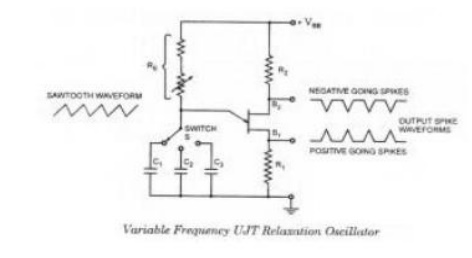Home | | Electronic Circuits II | UJT Relaxation Oscillator

# UJT Relaxation Oscillator

The relaxation oscillator shown in figure consists of UJT and a capacitor C which is charged through resistor RE when inter base voltage VBB is switched on.

UJT Relaxation Oscillator

The relaxation oscillator shown in figure consists of UJT and a capacitor C which is charged through resistor RE when inter base voltage VBB is switched on. During the charging period, the voltage across the capacitor increases exponentially until it attains the peak point voltage VP.

When the capacitor voltage attains voltage VP, the UJT switches on and the capacitor C rapidly discharges through B1. The resulting current through the external resistor R develops a voltage spike, as illustrated in figure and the capacitor voltage drops to the value VV.

The device then cuts off and the capacitor commences charging again. The cycle  is  repeated continually generating a saw-tooth waveform across capacitor C. The resulting waveforms of capacitor voltage VC and the voltage across resistor R (VR) are shown in figure. The frequency of the input saw- tooth wave can be varied by varying the value of resistor RE as it controls the time constant (T = REC) of the capacitor charging circuit.

The discharge time t2 is difficult to calculate because the UJT is in its negative resistance region and its resistance is continually changing. However, t2 is normally very much less than t1 and can be neglected for approximation.

For satisfactory operation of the above oscillator the following two conditions for the turn-on and turn-off of the UJT must be met.

RE < VBB – VP / IP and RE > VBB – VV / IV

That is the range of resistor RE should be as given below

VBB – VP / IP > RE >  VBB – VV / IV

The time period and, therefore, frequency of oscillation can be derived as below. During charging of capacitor, the voltage across the capacitor is given as

Vc = VBB(l-e-/ReC)

where REC is the time constant of the capacitor charging circuit and t is the time from the commencement of the charging.The discharge of the capacitor commences at the end of charging period t1 when the voltage across the capacitor Vc becomes equal to VP, that is, (ȠVBB + VB)

VP = ȠVBB + VB = VBB(l-e-/ReC) Neglecting VB in comparison to ȠVBB we have

ȠVBB = VBB(l-e-1/ReC)

Or e-t1/ReC = 1 – Ƞ

So charging time period, t1 = 2.3 RE C log10 1/1- ȠSince discharging time duration t2 is negligibly small as compared to charging time duration t1 so taking time period of the wave, T = t1

Time period of the saw-tooth wave, T = 2.3 RE C log10 1/1- Ƞ and frequency of oscillation f = 1/T = 1/2.3REClog10 (1-Ƞ)

By including a small resistor in each base circuit, three useful outputs (saw- tooth waves, positive triggers, and negative triggers), as shown in figure, can be obtained. When the UJT fires, the surge of current through Bt causes a voltage drop across R1 and produces the positive going spikes.

Also  at  the  UJT  firing         time,  the  fall of  VEB causes IB to  rise  rapidly  and generate the negative-going spikes across R2, as shown in figure. R1 and R2 should be much smaller than RBB to avoid altering the firing voltage of the UJT.

A wide range of oscillation frequencies can be achieved by making RE adjustable and including a switch to select different values of capacitance, as illustrated. As already mentioned in previous blog post there is upper and lower limits to the signal source resistance RE for the satisfactory operation of the UJT.

Study Material, Lecturing Notes, Assignment, Reference, Wiki description explanation, brief detail
Electronic Circuits : Blocking Oscillators and Timebase Generators : UJT Relaxation Oscillator |